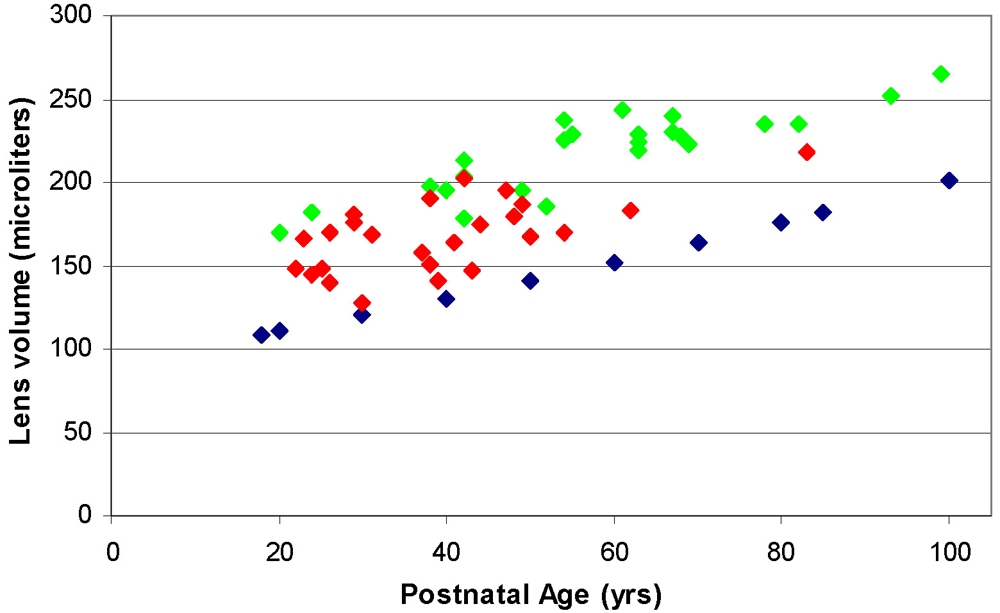Figure 3 of Augusteyn, Mol Vis 2008; 14:90-94.

Figure 3. Comparison of the volumes of fixed and unfixed lenses. The volumes of fixed (blue diamond)  and unfixed (green diamond)  in vitro lenses and fully accommodated in vivo lenses (red diamond)  are shown. Volumes of unfixed lenses were calculated using the published dimensions for individual lenses, assuming an ellipsoid shape. Fixed lens volumes were calculated using values calculated from the published regression equations for thickness and diameter .